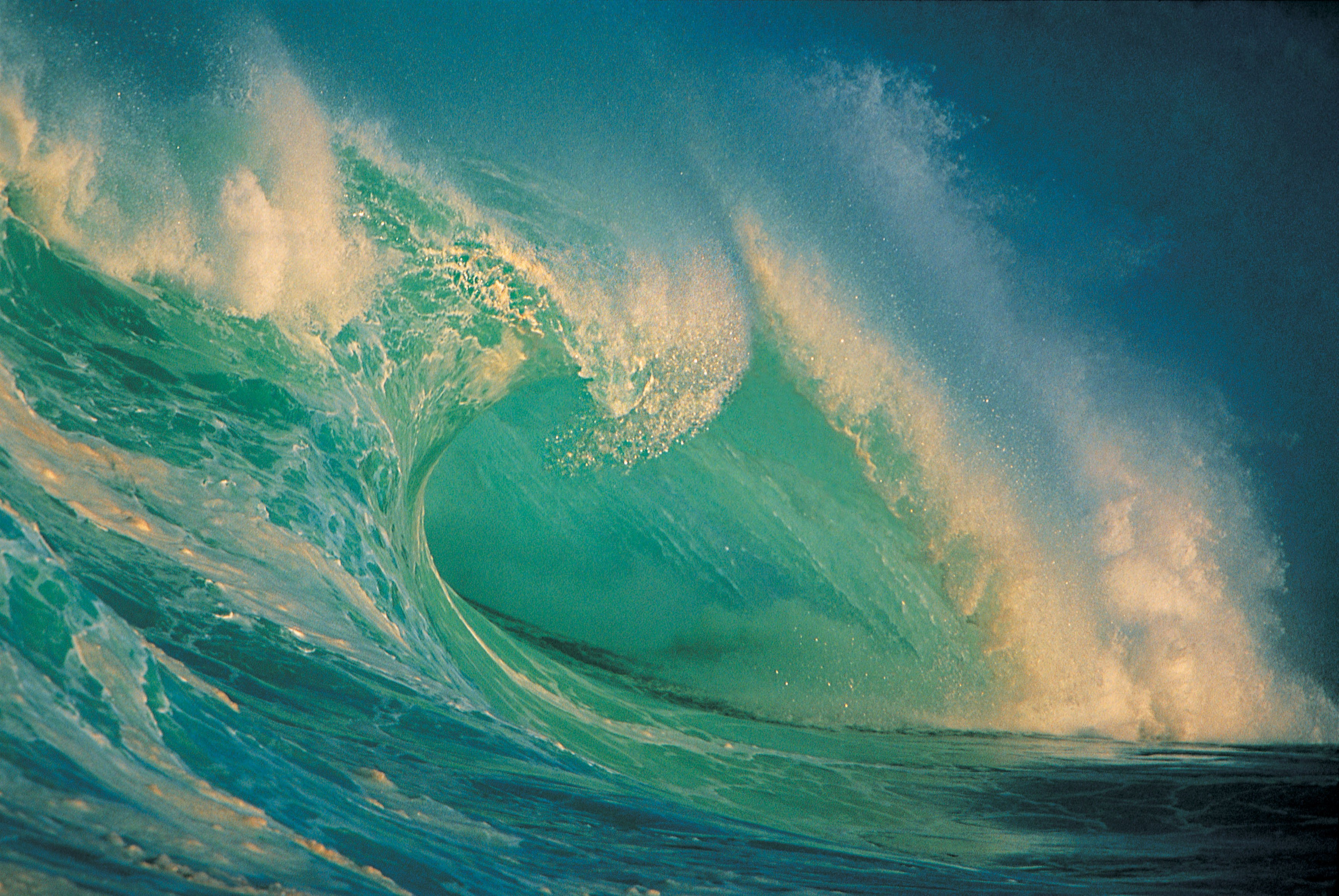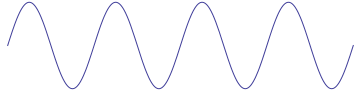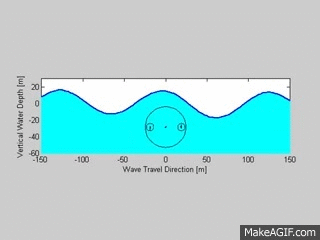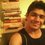# Mathematics of Ocean WavesOcean waves - magnificent cresting forms seem to personify creatures of the sea as they swell, roll and break on shore. Sophisticated mathematical equations and properties have been developed over the centuries to describe them. To understand and explore some of the mathematics that is used to explain their varying shapes, sizes, forms and idiosyncrasies, a look at the general nature of waves is needed.

Consider two people creating waves by undulating a rope between them. Imagine the wave traveling through the rope. The rope is not moving closer to either person. What is being transported between the two people is energy. Thus, waves are motions that carry energy over a medium. In this case the medium is a rope. It may be water (ocean waves), the earth (seismic earthquake waves), electromagnetic field (radio waves), air (sound waves). Waves are created when the medium is disturbed or agitated in some way. Ocean waves are created when either the wind, an earthquake, an object (such as a moving boat) and/or gravitational pull of the moon and the sun (causing tides) disturb the water (the medium). Ocean waves travel on the surface of the water. These undulating forms have a somewhat random quality to them when there are multiple disturbances.

Much study was generated in the $1800's$ on the mathematics of ocean waves. Observations at sea and in controlled laboratory experiments helped scientists arrive at interesting conclusions. It started in Czechoslovakia in $1802$, when Franz Gertner formulated the initial wave theory. In his observations he wrote how water particles in a wave move in circles. The water in the crest of the wave moves in the direction of the wave and that in the trough (the lowest point of the wave) moves in the opposite direction. On the surface, each water particle moves in a circular orbit before returning to its original position. This circle was found to have a diameter equal to the height of the wave. There are circles being generated by the particles throughout the depth of the water. But the deeper the particle the smaller its circle. In fact, it was found that at a depth of $\dfrac{1}{9}$ the wave length, the diameter of the circular orbit is about half that of the circular orbit of a surface particle.

Since waves are tied into these circling particles and since sinusoidal and cycloid shaped curves also depend on rotating circles, it is not surprising that these mathematical curves and their equations are used in the description of ocean waves. But it was discovered that ocean waves are not strictly sinusoidal or any other purely mathematical curve. The depth of the water, the intensity of the wind, the tides are only some of the variables that must be considered when describing waves. Today ocean waves are studied by applying the mathematics of probability and statistics. A large number of small waves are considered and predictions are formulated from the data collected.

$\text{Some other interesting mathematical properties of ocean waves are:}$

$\bullet$ The wave length depends upon the period.

$\bullet$ The wave height does not depend on the period or the length ( there are some exceptions in which the influence of the period and the length are minute).

$\bullet$The wave will break when the angle of the crest exceeds $120^{\circ}$. When the wave breaks most of its energy is then consumed.

$\bullet$ Another way to determine when the waves will break is to compare its height with its length. When this ratio is greater than $\dfrac{1}{7}$, the wave will break.

$\huge \text{Some Definitions}$

Crest : the highest point of the wave.

Trough : the lowest point of the wave.

Wave Height : The vertical distance from the crest to the trough.

Wave Length : The horizontal distance between two consecutive crests.

Wave Period : The time in seconds it takes a wave crest to travel one wave length.

Sinusoidal Curve is of the shape V - - which is a periodic (regularly repeats its shape) trigonometric function. Here is the graph looks like :Cycloid Curve is the curved which is the path of a point on a circle that is rolling along a straight line. Here is the graph looks like :Here is the superposition of the above two curves looks like :Credits : More Joy of Mathematics !

Leave your amazing comments & ideas here. and Keep Exploring the Universe of Mathematics.Note by Sandeep Bhardwaj
6 years, 2 months ago

This discussion board is a place to discuss our Daily Challenges and the math and science related to those challenges. Explanations are more than just a solution — they should explain the steps and thinking strategies that you used to obtain the solution. Comments should further the discussion of math and science.

When posting on Brilliant:

• Use the emojis to react to an explanation, whether you're congratulating a job well done , or just really confused .
• Ask specific questions about the challenge or the steps in somebody's explanation. Well-posed questions can add a lot to the discussion, but posting "I don't understand!" doesn't help anyone.
• Try to contribute something new to the discussion, whether it is an extension, generalization or other idea related to the challenge.

MarkdownAppears as
*italics* or _italics_ italics
**bold** or __bold__ bold
- bulleted- list
• bulleted
• list
1. numbered2. list
1. numbered
2. list
Note: you must add a full line of space before and after lists for them to show up correctly
paragraph 1paragraph 2

paragraph 1

paragraph 2

[example link](https://brilliant.org)example link
> This is a quote
This is a quote
    # I indented these lines
# 4 spaces, and now they show
# up as a code block.

print "hello world"
# I indented these lines
# 4 spaces, and now they show
# up as a code block.

print "hello world"
MathAppears as
Remember to wrap math in $$ ... $$ or $ ... $ to ensure proper formatting.
2 \times 3 $2 \times 3$
2^{34} $2^{34}$
a_{i-1} $a_{i-1}$
\frac{2}{3} $\frac{2}{3}$
\sqrt{2} $\sqrt{2}$
\sum_{i=1}^3 $\sum_{i=1}^3$
\sin \theta $\sin \theta$
\boxed{123} $\boxed{123}$

Sort by:

Are you sure that is a cycloid curve?

According to the definition, this is a cycloidal curve:What you posted is a superposition of those two, really

- 6 years, 2 months ago

Thank you Agnishom, I've updated it.

- 6 years, 2 months ago

What about waves that roll onto themselves? Like the one shown in the picture at the top of the page.

- 1 year, 7 months ago